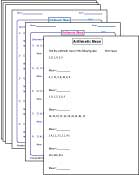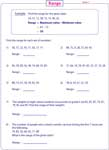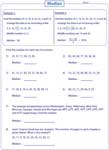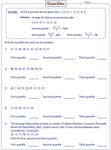1. Worksheets>
2. Math>
3. Statistics>
4. Mean, Median, Mode and Range

# Central Tendency Worksheets: Mean, Median, Mode and Range

Mean, median, mode and range worksheets contain printable practice pages to determine the mean, median, mode, range, lower quartile and upper quartile for the given set of data. Interesting word problems are included in each section.

Finding Average

Average or mean worksheets have plentiful exercises to find the average of numbers, numbers with practical units and decimals.Mean (or Average) Worksheets

Finding Range

Identify the maximum and minimum values to find the range of the given data. Word problems are included for practice.Finding Median

In these worksheets, identify the median (middle value) of the represented data. Two word problems included.Finding Quartiles

Determine the first (lower) quartile, second (median) quartile and the third (upper) quartile of the given data. One word problem included.Mean, Median, Mode and Range: Level 1

These central tendency worksheets contain a mixed review of mean, median, mode and range concepts. Around 8 data are used in level 1.Mean, Median, Mode and Range: Level 2

Find the mean, median, mode and range of each set of data. Each sheet has six problems with around 15 data.Word Problems: Level 1

This exclusive section has five word problems to find the mean, median, mode and range of the given data.Word Problems: Level 2

A variety of informative data are included as word problems in these central tendency worksheets to determine the values of mean, median, mode and range.The entire collection of worksheets on mean, median, mode and range can be downloaded in a jiffy!

Related Worksheets